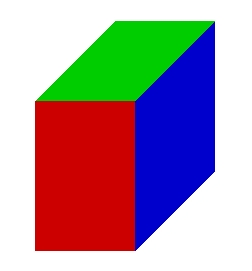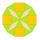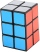# Minimum surface

Find the length, breadth, and height of the cuboid-shaped box with a minimum surface area, into which 50 cuboid-shaped blocks, each with length, breadth, and height equal to 4 cm, 3 cm, and 2 cm respectively, can be packed.

x =  12 cm
y =  10 cm
z =  10 cm

### Step-by-step explanation:Did you find an error or inaccuracy? Feel free to write us. Thank you!Tips to related online calculators
Tip: Our volume units converter will help you with the conversion of volume units.

## Related math problems and questions:

• Three cubesTwo cube-shaped boxes with edges a = 70 cm; b = 90 cm must be replaced by one cube-shaped box. What will be its edge?
• Prism XThe prism with the edges of the lengths x cm, 2x cm, and 3x cm has volume 20250 cm3. What is the area of the surface of the prism?
• Cube-shaped boxDesign the size of the smallest possible cube-shaped box where three types of 3cm, 5cm, 6cm small cubes could be stacked to make full use of the box space (each type of cube separately). Can you find out how many smallest cubes are in the box?
• Surface of cubesPeter molded a cuboid 2 cm, 4cm, 9cm of plasticine. Then the plasticine split into two parts in a ratio 1:8. From each piece made a cube. In what ratio are the surfaces of these cubes?
• CuboidThe volume of the cuboid is 245 cm3. Each cuboid edge length can be expressed by an integer greater than 1 cm. What is the surface area of the cuboid?
• Cuboid - ratiosThe sizes of the edges of the cuboid are in the ratio 2: 3: 5. The smallest wall has an area of 54 cm2. Calculate the surface area and volume of this cuboid.
• Two boxes-cubesTwo boxes cube with edges a=38 cm and b = 81 cm is to be replaced by one cube-shaped box (same overall volume). How long will be its edge?
• Area of a cubeCalculate the surface area of a cube if its volume is equal to 729 cubic meters.
• Cube surface and volumeThe surface of the cube is 500 cm2, how much cm3 will be its volume?
• Equilateral cylinderEquilateral cylinder (height = base diameter; h = 2r) has a volume of V = 199 cm3 . Calculate the surface area of the cylinder.
• Cuboid and ratioFind the dimensions of a cuboid having a volume of 810 cm3 if the lengths of its edges coming from the same vertex are in ratio 2: 3: 5
• Volume and areaWhat is the volume of a cube which has an area of 361 cm2?
• The volume 2The volume of a cube is 27 cubic meters. Find the height of the cube.
• Cuboid edgesThe lengths of the cuboid edges are in the ratio 2: 3: 4. Find their length if you know that the surface of the cuboid is 468 m2.
• Diameter = heightThe cylinder's surface, the height of which is equal to the diameter of the base, is 4239 cm square. Calculate the cylinder volume.
• Cube volumeThe cube has a surface of 384 cm2. Calculate its volume.
• Cube surfce2volumeCalculate the volume of the cube if its surface is 150 cm2.